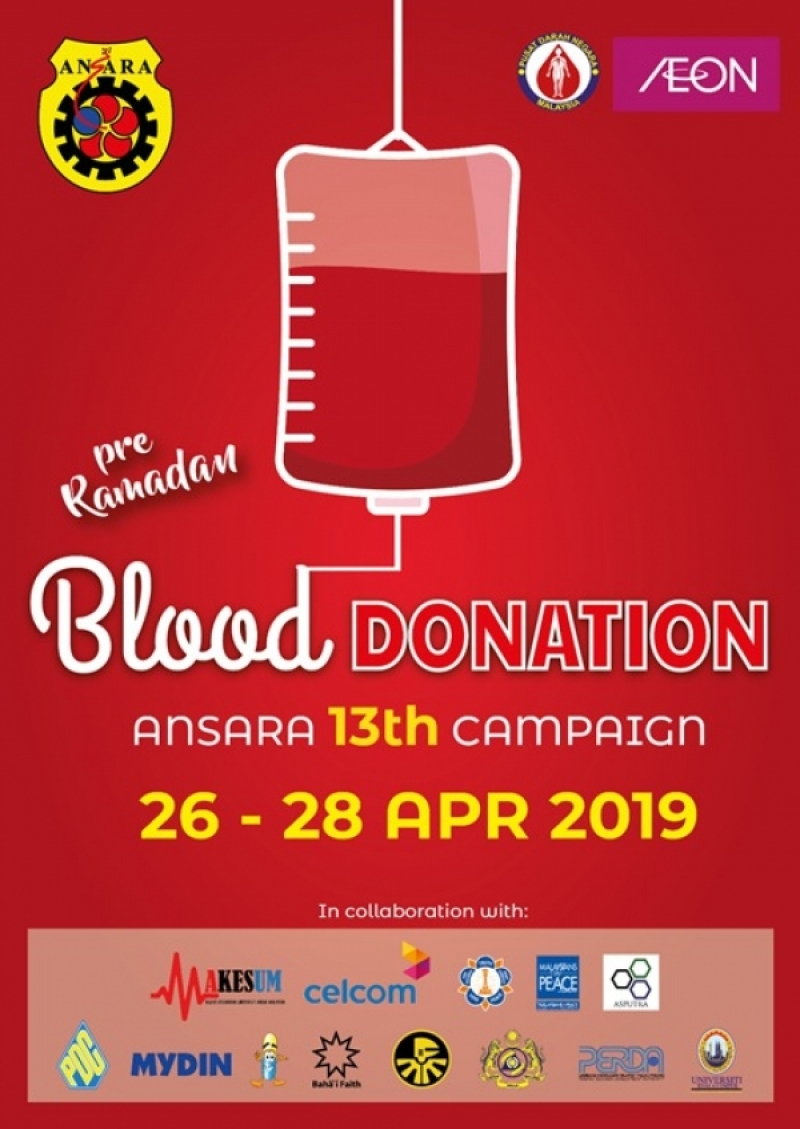# ANSARA PRA-RAMADAN BLOOD DONATION CAMPAIGN 2019 REPORT

Posted By AHMAD NAZMI AB RAZAK, May 02 2019ANSARA PRA-RAMADAN BLOOD DONATION CAMPAIGN 2019 (ABDC 2019)

As at 30/4/2019 :

KUALA LUMPUR & SELANGOR
1. AEON Mall AU2 Setiawangsa
26/4 = 18 units
27/4 = 43 units
28/4 = 37 units
Total = 98 units

2. AEON Mall Metro Prima, Kepong
26/4 = 38 units
27/4 = 62 units
28/4 = 98 units
Total = 198 units

3. AEON Taman Maluri Shopping Centre
27/4 = 49 units
28/4 = 52 units
Total = 101 units

4. Alpha Angle Shopping Centre, Wangsa Maju
26/4 = 11 units
27/4 = 36 units
28/4 = 44 units
Total = 91 units

5. Bukit Raja Shopping Centre
26/4 = 40 units
27/4 = 37 units
28/4 = 83 units
Total = 160 units

6. AEON Mall Bukit Tinggi
26/4 = 35 units
27/4 = 57 units
28/4 = 126 units
Total = 218 units

7. AEON Mall Cheras Selatan
27/4 = 74 units
28/4 = 110 units
Total = 184 units

8. AEON Mall Rawang
27/4 = 41 units
28/4 = 64 units
Total = 105 units

9. AEON Mall Shah Alam: 10am-6pm
26/4 = 43 units
27/4 = 97 units
28/4 = 109 units
Total = 249 units

10. AEON Taman Equine Shopping Centre: 10am-5pm
27/4 = 53 units
28/4 = 64 units
Total = 117 units

PERAK
11. Kinta City Shopping Centre
27/4 = 36 units
28/4 = 45 units
Total = 81 units

12. AEON Mall Ipoh Station 18
27/4 = 32 units
28/4 = 61 units
Total = 93 units

13. AEON Mall Ipoh Klebang
27/4 = 34 units
28/4 = 35 units
Total = 69 units

14. AEON Mall Sri Manjung
27/4 = 41 units
28/4 = 70 units
Total = 111 units

15. AEON Mall Taiping
27/4 = 31 units
28/4 = 43 units
Total = 74 units

NEGERI SEMBILAN
16. AEON Mall Seremban 2
27/4 = 93 units
28/4 = 165 units
Total = 258 units

17. AEON Nilai
26/4 = 64 units
27/4 = 103 units
28/4 = 124 units
Total = 291 units

MELAKA
18. AEON Mall Bandaraya Melaka
27/4 = 126 units
28/4 = 126 units
Total = 252 units

19. AEON Melaka Shopping Centre, Ayer Keroh
27/4 = 55 units

JOHOR
20. AEON Mall Tebrau City
27/4 = 75 units
28/4 = 103 units
Total = 178 units

21. AEON Mall Bandar Dato Onn
27/4 = 50 units

PENANG
22. AEON Mall Bukit Mertajam
26/4 = 39 units
27/4 = 74 units
28/4 = 97 units
Total = 210 units

KELANTAN
23. AEON Mall Kota Bharu
26/4 = 56 units
27/4 = 56 units
Total = 112 units

TERENGGANU
24. Mydin Gong Badak
26/4 = 51 units
27/4 = 93 units
28/4 = 76 units
Total = 220 units

PERLIS
25. UTC Perlis
26/4 = 25 units
27/4 = 30 units
28/4 = 5 units
Total = 60 units

SARAWAK
26. AEON Mall Kuching Central
26/4 = 14 units
27/4 = 23 units
Total = 37 units

Total 26/4 = 434 units
Total 27/4 = 1,501 units
Total 28/4 = 1,737 units
Total ABDC 26-28/4/2019 = 3,672 units

Total ABDC IPTA = 1,491 units (excl. 9 UA & other branches of UiTM)
Total ABDC others = 113 units (Amanjaya Mall Sg. Petani & PDC)

TOTAL ABDC as at 30/4/19 = 5,276 units

SUMMARY REPORT on ABDC2019:

� The overall collection as at 30/4 is 5,276 units @ 40 locations, 42% lower than overall 2018 (9,038 units) @ 67 locations

� 26 venues held for the weekends vs 28 venues in 2018

� Top 5 Performers:
1. Nilai (291 units, REMARKABLE 1st timer)
2. Seremban 2 (258 units)
3. Bandaraya Melaka (252 units, HIGHEST since 2011)
4. Shah Alam (249 units)
5. Gong Badak (220 units)

� 8 locations registered growth vs 2018: Bukit Mertajam (123%), Tebrau City (41%), Bandaraya Melaka (27%), PDC (22%), Sri Manjung (21%), Bukit Raja (20%), AU2 (16%) & KB (12%)

� 5 locations recorded more than past years average: Sri Manjung, Bandaraya Melaka, Bukit Mertajam (highest in 5 years), PDC (highest ever) & Gong Badak

� 3 new locations: Nilai (291 units), Amanjaya Mall Sg. Petani (53 units) & UTC Perlis (60 units)

� 12 locations from 11 universities (1,491 units): UPNM, UiTM, UKM, UPM, USM, UNIMAP, USIM, UTM, UTHM, UMP (2) & UNIMAS

� Min 25 more locations:
1. 2 May (today): Celcom HQ & Ayer Hangat Village, Langkawi (LADA)
2. 3 May: Aman Sentral
3. 4 May: Bukit Indah, Permas Jaya, TMG Mall Tanjung Lumpur
4. 14 Jun: Imago Mall, KK
5. 15 Jun: Mydin Bukit Mertajam
6. 18 Jun: Komtar
7. tbc Jun: UZMA Tower, Shaftsbury & more InsyaAllah
8. Min 10 venues from UIA, UM, UPSI, UUM, UTeM, UMK, UniSZA, UMT, UMS & UiTM Branches